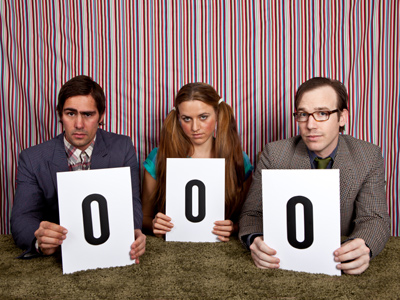0 lies between -1 and 1 on a numberline.

# Compare and Order Numbers 1

This Math quiz is called 'Compare and Order Numbers 1' and it has been written by teachers to help you if you are studying the subject at elementary school. Playing educational quizzes is an enjoyable way to learn if you are in the 3rd, 4th or 5th grade - aged 8 to 11.

It costs only \$12.50 per month to play this quiz and over 3,500 others that help you with your school work. You can subscribe on the page at Join Us

Being able to compare and order numbers is about knowing the size of numbers. Knowing 1 to 100 is straightforward, but what about negative numbers or numbers with decimal points? Is 0.7 larger than 0.07? What about larger numbers like 9,973 and 9,987 - which is larger? If you know your units tens, hundreds and thousands, along with your tenths, hundredths and thousandths, you should be able to work the answers out.

1.
Which number is fewer than 53?
45
54
68
55
I hope you got this one right!
2.
Which is the smallest number?
5,478
5,748
5,847
5,784
All of the numbers have 5 thousands but 5,478 only has 4 hundreds, while all the rest have 7 or 8
3.
Which number lies halfway between 1,020 and 1,050?
1,030
1,035
1,040
1,045
1,020 + 15 = 1,035 and 1,050 - 15 = 1,035 too
4.
Which is the longest distance?
9,412 m
9,214 m
9,421 m
9,241 m
9,421 and 9,412 both have 9 thousands and 4 hundreds but 9,421 has 2 tens while 9,412 only has 1
5.
Which of these numbers is the largest?
0.2
1.3
0.9
1.1
Decimals can be confusing. If two numbers share the same units (like 1.3 and 1.1) then the one with the highest tenths is higher
6.
What does this symbol mean < ?
Less than
Greater than
Equal to
Greater than or equal to
The smallest part of the symbol will be next to the smallest number e.g. 2 < 3
7.
What does this symbol mean >?
Less than
Greater than
Equal to
Greater than or equal to
The largest part of the symbol will be next to the largest number e.g. 3 > 2
8.
Which of these numbers is greater?
4,561
4,516
4,615
4,651
4,651 and 4,615 both have 4 thousands and 6 hundreds but 4,651 has 5 tens while 4,615 only has 1
9.
Which number comes after -4 on a number line?
-2
-3
-5
-1
A number line with negative numbers would be -5, -4, -3, -2, -1
10.
Which number comes next? -3, -2, -1
0
1
2
3
0 is between -1 and 1
Author:  Amanda Swift